BetMGM Championship Odds
+250
2.5 to 1Nets
23.7% implied probability

+500
5 to 1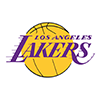Lakers
13.8% implied probability

+750
7.5 to 1Bucks
9.7% implied probability

+1000
10 to 1Warriors
7.5% implied probability

+1300
13 to 1Jazz
5.9% implied probability

+1300
13 to 1Suns
5.9% implied probability

+1600
16 to 1Clippers
4.9% implied probability

No changes have been recorded yet.
+2000
20 to 1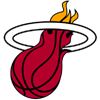Heat
3.9% implied probability

+2000
20 to 176ers
3.9% implied probability

+2200
22 to 1Nuggets
3.6% implied probability

No changes have been recorded yet.
+3000
30 to 1Mavericks
2.7% implied probability

No changes have been recorded yet.
+4000
40 to 1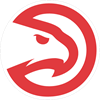Hawks
2.0% implied probability

+4000
40 to 1Bulls
2.0% implied probability

+4000
40 to 1Celtics
2.0% implied probability

No changes have been recorded yet.
+6600
66 to 1Trail Blazers
1.2% implied probability

+8000
80 to 1Grizzlies
1.0% implied probability

+8000
80 to 1Knicks
1.0% implied probability

No changes have been recorded yet.
+10000
100 to 1Pacers
0.8% implied probability

No changes have been recorded yet.
+12500
125 to 1Pelicans
0.7% implied probability

+12500
125 to 1Hornets
0.7% implied probability

+15000
150 to 1Raptors
0.5% implied probability

No changes have been recorded yet.
+15000
150 to 1Wizards
0.5% implied probability

No changes have been recorded yet.
+25000
250 to 1Timberwolves
0.3% implied probability

No changes have been recorded yet.
+25000
250 to 1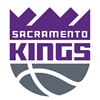Kings
0.3% implied probability

No changes have been recorded yet.
+30000
300 to 1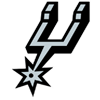Spurs
0.3% implied probability

No changes have been recorded yet.
+50000
500 to 1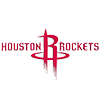Rockets
0.2% implied probability

No changes have been recorded yet.
+50000
500 to 1Thunder
0.2% implied probability

No changes have been recorded yet.
+50000
500 to 1Cavaliers
0.2% implied probability

No changes have been recorded yet.
+50000
500 to 1Pistons
0.2% implied probability

No changes have been recorded yet.
+50000
500 to 1Magic
0.2% implied probability

No changes have been recorded yet.